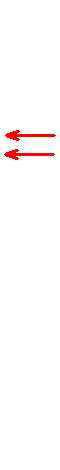Americans For Tax Reform Website, www.atr.org

Enter the value and click CalculateHow This Calculator Works The ATR 2008 401(k) Calculator attempts to show how various changes to the tax structure will affect the underlying value of your 401(k).  Since taxes are a cost drag on investments, raising or lowering key tax rates has the effect of raising or lowering the value of your nest egg—regardless of whether that nest egg is in a tax-deferred account or not.  When the stock market goes up and down, so does your 401(k). Below are the inputs for each of the scenarios.  The calculation’s estimates were prepared by Rutledge Capital. Current Law: When you input the value of your 401(k) today, implicit in that value are the effects of current tax law.  These include a 15% capital gains rate, a 15% dividends rate, a 35% corporate income tax rate, and depreciation of capital equipment Obama: The Obama tax plan assumes a 20% capital gains rate, a 20% dividends rate, a 35% corporate income tax rate, and depreciation of capital equipment McCain: The McCain tax plan assumes a 15% capital gains rate, a 15% dividends rate, a 25% corporate income tax rate, and expensing of capital equipment Hill Dems: The Hill Democrat tax plan assumes a 28% capital gains rate, a 39.6% dividends rate, a 35% corporate income tax rate, and depreciation of capital equipment ATR: The ATR tax plan assumes a 0% capital gains rate, a 0% dividends rate, a 25% corporate income tax rate, and expensing of capital equipment